Tonne (T) As A Unit For Measuring Mass  by  Prisca Mwikya

6

Units of mass

 Units Symbol Grams g Kilograms kg Tonnes T

Which units would you use to weigh you exercise books?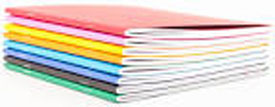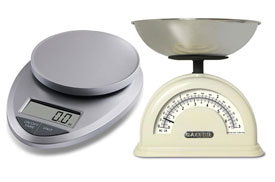Which units are used to measure average objects like the cylinders below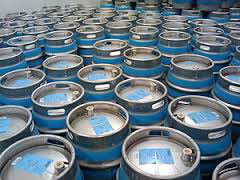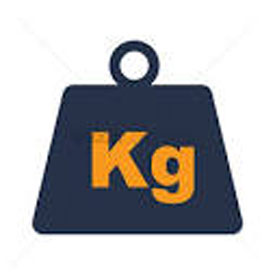Kilogrammes are used to weigh objects which are not light and not very heavy

Which unit do we use to weigh very heavy objects like the one below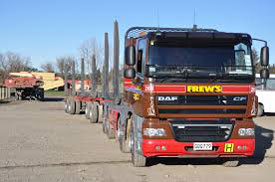We use tonnes (t) to weigh very heavy objects

1. How many grams make 1kg
2. How many kg make 1T
3. How many grams make 1T
4. 1kg = 1000g
5. 1T = 1000kg
6. 1T = 1000,000g

1.The mass of your class senator is 50,000g what is his mass in kg

1000g = 1kg

50000g = 50,000

1000

= 50kg

2.The mass of a pickup full of sand is 6000kg what is its mass in tonnes?

1000kg = 1T

6000kg = 6000

1000

= 6T

3.How many tonnes are there in the following

1.8,700kg

2.8,500kg

3.289,560kg

4.546,000kg

5.4,562kg